# Implementation of a lattice Boltzmann method for immiscible two-phase flow simulations using the level set method

Supervision: Dipl.-Math. Regina Ammer (CE)

We implemented a lattice Boltzmann method for immiscible multiphase flow simulations combined with the level set method according to the paper of G. Thömmes et al. . The level set method is used to compute the movement of the interface between the phases. The input for the level set method is computed by the lattice Boltzmann method. We verified our implementation with different test cases.

### Motivation

In engineering the immiscible multiphase flow problem often needs to be considered. For example, bubble dynamics are decisive for the design of chemical reactors. Also fingering in oil recovery is a common multiphase problem.

We restricted ourselves to two fluid phases, but the method is trivially extensible for multiple phases. There are different approaches to solve the immiscible two-phase problem. The most spread among these is the colour gradient method of Gunstensen and Rothman. The downside of this method is that it is only applicable for small density and viscosity differences. Another approach is the concept of interaction potentials by Shan and Chen which actually models miscible fluids and can only approximately describe the behavior of immiscible fluids. Furthermore, there are free surface methods which only take one fluid into account for computation . They work best for large viscosity and density ratios.

In our project we used a coupled lattice Boltzmann level set method. The motion of the interface is modeled by the level set method. The input data for the level set method is given by the lattice Boltzmann method (LBM), which is applied independently for each fluid phase. This method has the advantage that it allows large ranges of viscosity- and density-ratios between the fluid phases, while describing a sharp interface.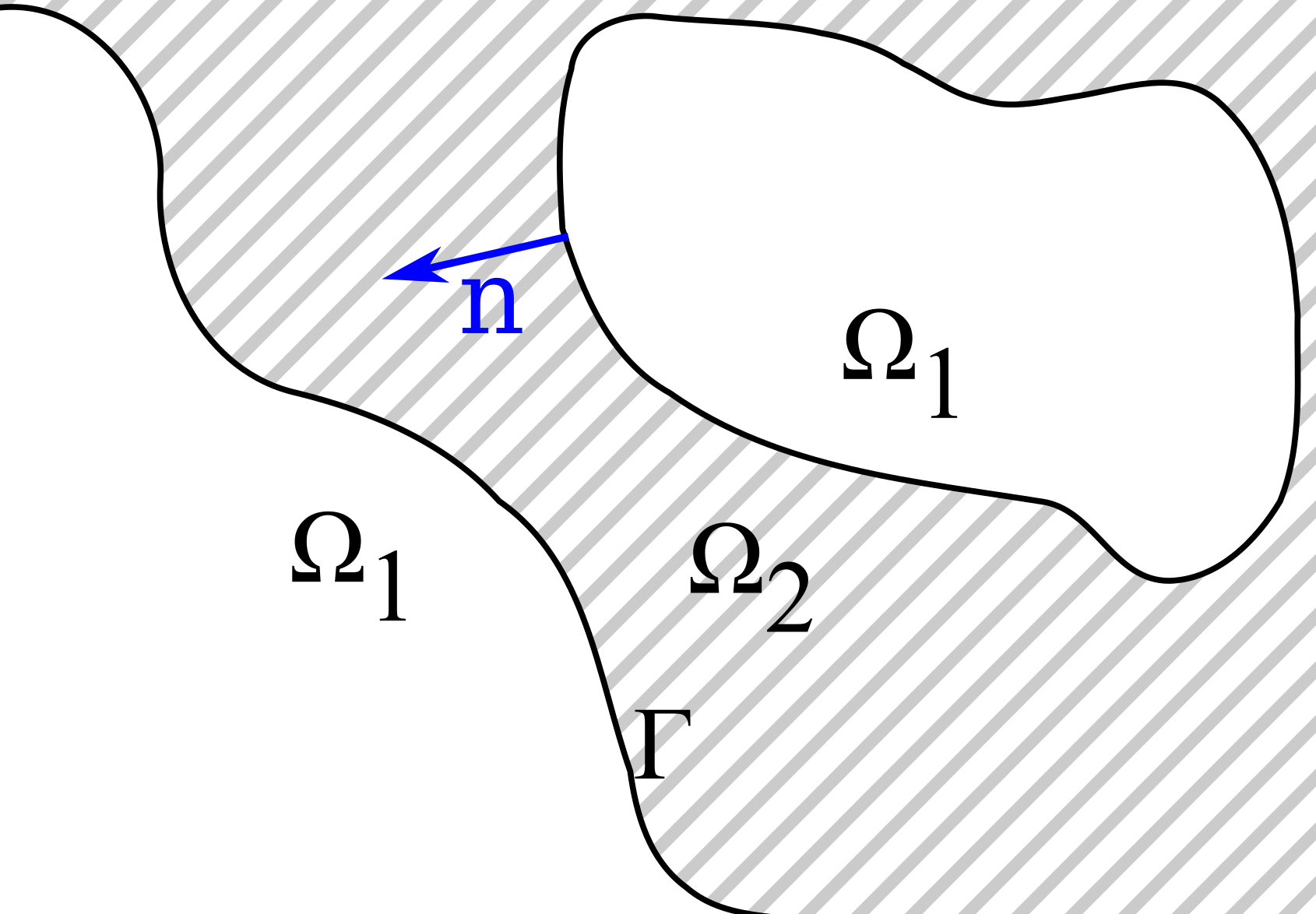Figure 1: The mathematical description of the two fluid domains

### Methods

#### Macroscopic laws

The incompressible two-phase flow problem can be described mathematically with the Navier-Stokes equations for two incompressible fluids (eqs. (1.1) and (1.2), see ). To apply them, we distribute our domain into three parts: Ω1 and Ω2 describe the two fluids and Γ is the interface in-between (Fig. 1). The material properties in each fluid are constant and the interface is assumed to be sharp. Thus, the overall material properties are discontinuous and the fluids will not mix.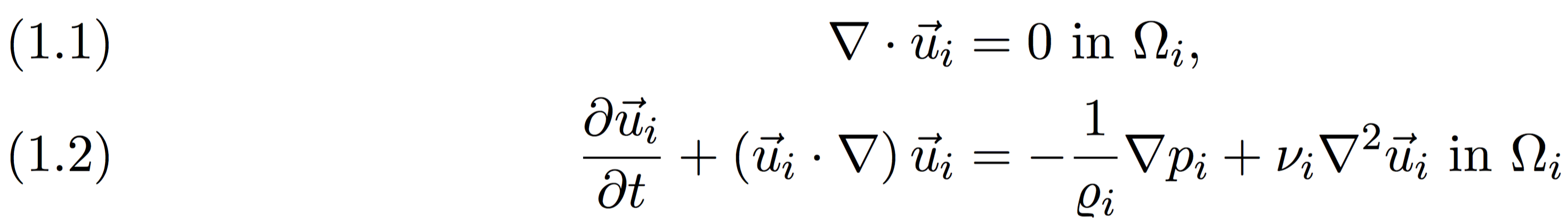Here, ui is the velocity, pi the pressure, νi the kinematic viscosity and ρi the mass density. As boundary conditions and initial conditions we use,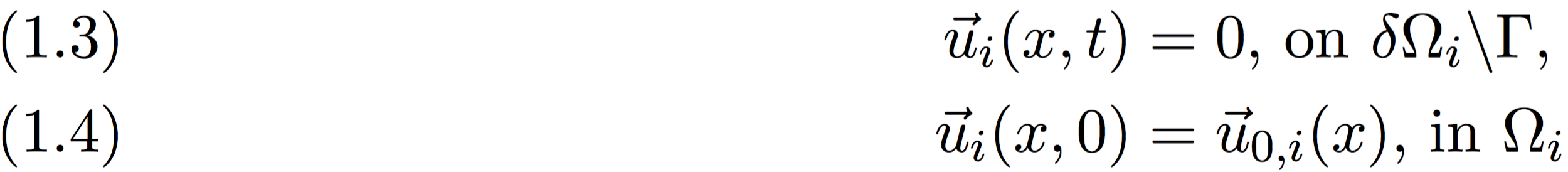At the interface Γ we have a no-slip condition, i.e. continuity of the velocities between the fluid phases and the balance of viscous shear stress with surface tension. Therefore, we get the jump conditions,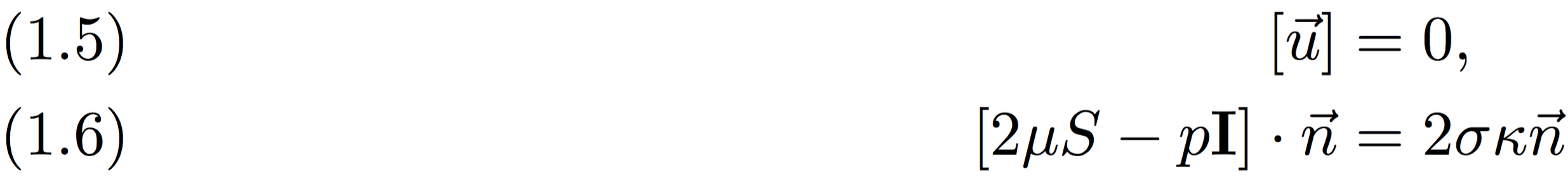#### Numerical methods

The Navier-Stokes equations are solved with a lattice Boltzmann method. A detailed introduction into the LBM by Succi can be found in . The position of the phase interface is tracked with a level set method, see e.g.  for a general introduction.

To ensure the macroscopic jump condition at the phase interface an additional boundary condition is introduced in the lattice Boltzmann method in neighbour-cells to the interface. In simplified terms, this condition is a bounce-back condition that ensures the continuity of velocities, as well as the balance of shear stress w.r.t. to the interface position between the LBM cells.
Moreover, a refill method is required to estimate the unknown PDFs of the LBM cells that changed their type from one fluid phase to the other, due to movement of the interface. Here, a weighted interpolation from three interior neighbour cells is used.

### Results

The algorithm was implemented in MATLAB-code where a level set library was used.
To assess the physical correctness, we used a couette flow scenario. Here, two layers of fluids are stacked upon another and a tangential velocity is induced at the top. The bottom of the domain was at rest and periodic boundaries were applied in horizontal direction. For this scenario an analytic solution to the Navier-Stokes equations is known. The velocity profile will have a kink at the phase interface corresponding to the ratio of the dynamic viscosites of the two fluid phases. See figure 3 for the comparison of numerical and analytic results.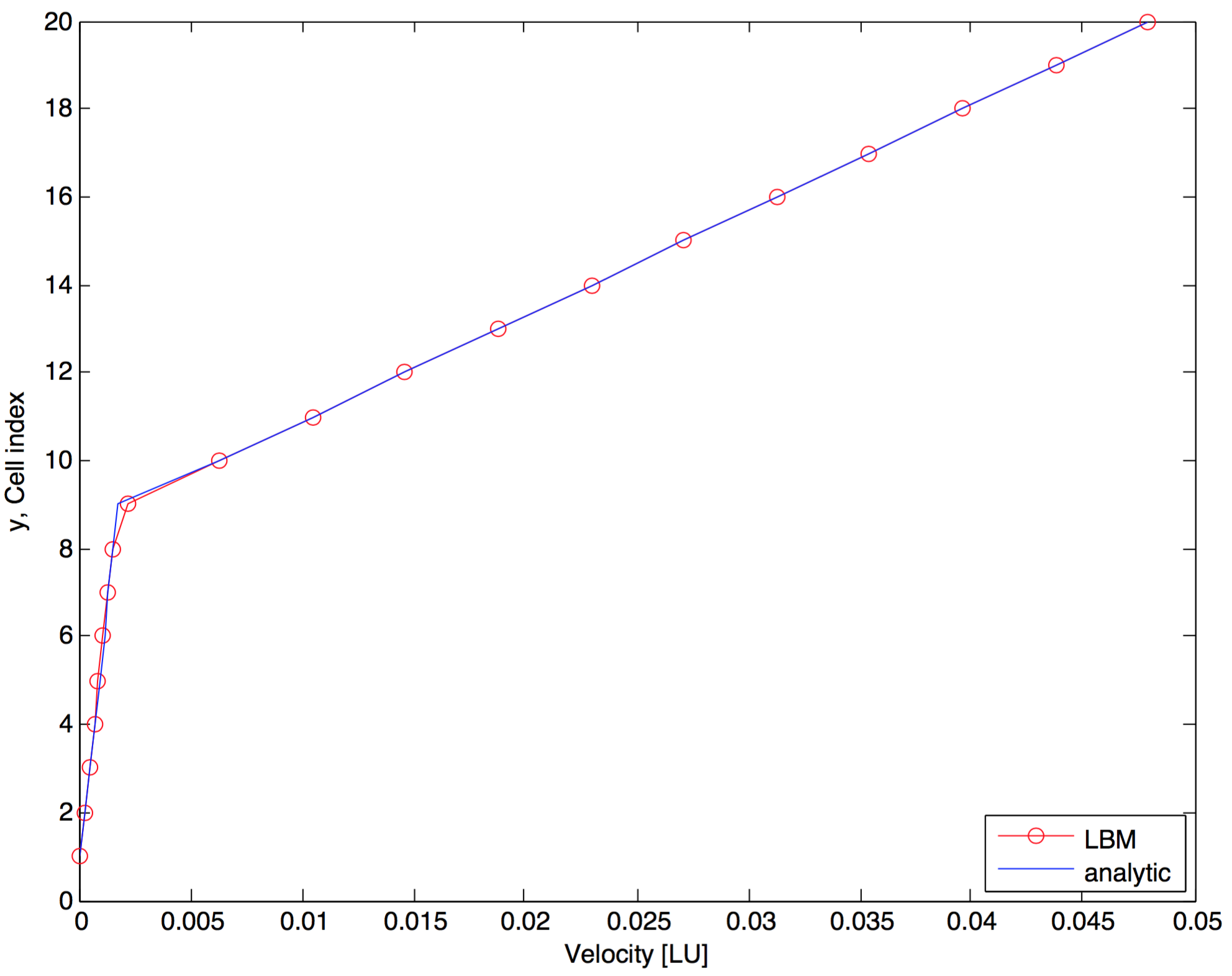Figure 3: The analytical and numerical velocity profile of two fluids in a couette flow. The upper layer had a viscosity of 0.5, the lower one a viscosity of 10

A relative error in the order of O(10-4) was achieved after 2000 LBM iterations for this setup and various other viscosity ratios and interface positions were also verified successfully.

Furthermore, we used a spherical bubble of one fluid phase immersed in the other one. For this scenario the pressure drop along the bubble surface due to surface tension is known. We were also able to successfully verify our simulations with this setup.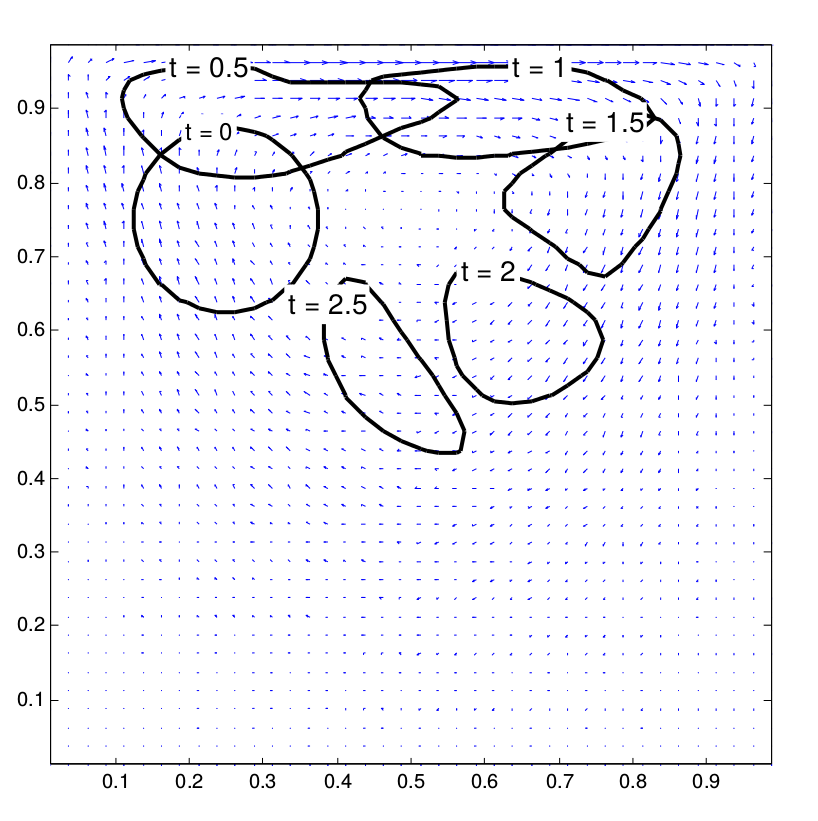Figure 4: The transient deformation of a drop inside a lid driven cavity flow

### Conclusion

We implemented a hybrid lattice Boltzmann method that uses the level set method to model the movement of the interface. We adapted the formulas given in  to fit in our environment. Finally, we verified our implementation with different test cases. It is a known problem that level set methods tend to suffer from mass losses , but still a good insight into the detailed flow behavior is possible. According, a variety of two-phase flow scenarios is possible for simulation with the presented method.

### References

 G. Thömmes, J. Becker, M. Junk, A.K. Vaikuntam, D. Kehrwald, A. Klar, K. Steiner, A. Wiegmann, A lattice Boltzmann method for immiscible multiphase flow simulations using the level set method, J. Comput. Phys. 228 (2009), 1139-1156.
 C. Körner, M. Thies, T. Hofmann, N.Thurey, U. Rüde, Lattice Boltzmann Model for Free Surface Flow for Modeling Foaming, J. Stat. Phys. 121 (2005) 179-196.
 J. FERZIGER, M. PERIC., Computational methods for fluid dynamics. Springer Science & Business Media, ISBN 978-3540420743 (2012).
 S. SUCCI, The Lattice Boltzmann Equation for Fluid Dynamics and Beyond, Clarendon Press, Oxford, ISBN 978-0-19-850398-9 (2001).
 S. OSHER, J. SETHIAN, Fronts propagating with curvature-dependent speed: algorithms based on Hamlton-Jacobi formulations, J. Comput. Phys. 79 (1988) 12-49.